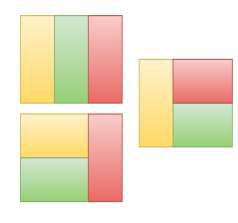# Count the number of ways to tile the floor of size n x m using 1 x m size tiles in C++

Given two numbers n and m representing the length and breadth of the floor of a room. The goal is to count the number of ways in which this floor can be tiled using the tiles of size 1Xm.

For Example

## Input

n=3 m=2

## Output

Count the number of ways to tile the floor of size n x m using 1 x m size tiles
are: 3

## Explanation

The ways will be three 1x2 tiles arranged as shown below −## Input

n=3 m=3

## Output

Count the number of ways to tile the floor of size n x m using 1 x m size tiles
are: 2

## Explanation

The ways will be three 1x3 tiles arranged vertically and horizontally. Only
two ways.

Approach used in the below program is as follows

In this approach we check the value of n. If n is less than m and has value 1 then there will be only one tile to be placed all over the floor of size 1Xm.

If n is equal to m then there will be 2 ways, placing n 1xm tiles all vertically and all horizontally. If n is greater than m then we will calculate ways using previous ways − ways[n−1]+ways[m−1].

• Take integers m and n for the dimensions of the floor and tiles.

• Function ways_tile_floor(int N, int M) takes the dimensions and returns the number of ways to tile the floor of size n x m using 1 x m size tiles.

• Take an array arr[ ] of length N+1 to store count of tiles for index i=current value of N.

• For arr tiles will be 0. So arr=0.

• Traverse arr[] using for loop from i=1 to i=N. For each arr[i], if i>M then calculate count using previous values. arr[i]=arr[i − 1] + arr[i − M].

• In case i=1 and or i<M, set arr[i] with 1.

• In case i=M, set arr[i]=2.

• At the end of the for loop, arr[N] will have a count of ways of arranging tiles.

• Return arr[N] as result.

## Example

Live Demo

#include <bits/stdc++.h>
using namespace std;
int ways_tile_floor(int N, int M){
int arr[N + 1];
arr = 0;
for (int i = 1; i <= N; i++){
if (i > M){
arr[i] = arr[i − 1] + arr[i − M];
}
else if (i < M || i == 1){
arr[i] = 1;
} else {
arr[i] = 2;
}
}
return arr[N];
}
int main(){
int n = 3, m = 2;
cout<<"Count the number of ways to tile the floor of size n x m using 1 x m size tiles are: "<<ways_tile_floor(n, m);
return 0;
}

## Output

If we run the above code it will generate the following output −

Count the number of ways to tile the floor of size n x m using 1 x m size tiles are: 3